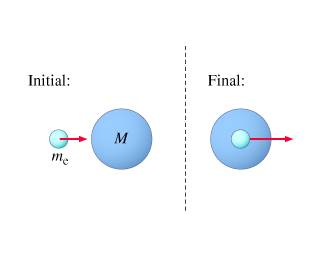# Inelastic collision energy relationship

## Homework Statement

An atom of mass M is initially at rest, in its ground state. A moving (nonrelativistic) electron of mass me collides with the atom. The atom+electron system can exist in an excited state in which the electron is absorbed into the atom. The excited state has an extra, "internal," energy E relative to the atom's ground state.

Find the kinetic energy that the electron must have in order to excite the atom.

## Homework EquationsInelastic collision:
m1*v1 + m2*v2 = (m1+m2)Vf

and possibly:

$$\Delta$$K = Ki - Kf

## The Attempt at a Solution

What i've got so far:
m1*v1 + m2*v2 = (m1+m2)Vf -->

me*ve + (0) = (me + M)*Vf -->

Vf = (me*ve)/(me + M)

$$\Delta$$K = Ki - Kf -->

Kf = (1/2)(me + M) * [(meve)/(me + M)]^2 --> simplified -->

(me*ve)^2/2(me+M)

Ki = just the Kinetic energy of the electron = (1/2)(me*ve^2 --> so..

(me*ve)^2/2(me+M) - (1/2)(me*ve^2) = E(?.. an assumption) --->

so K_e = (m_e * v_e)^2/2(m_e + M) - E --->

there's the problem, i have v_e in my solution. But first i need to know i'm on the right track which i think i am. Any advice?

Last edited:

Doc Al
Mentor
(me*ve)^2/2(me+M) - (1/2)(me*ve^2) = E(?.. an assumption) --->
You want K_i - K_f = E, since kinetic energy is lost to internal energy.

so K_e = (m_e * v_e)^2/2(m_e + M) - E --->

there's the problem, i have v_e in my solution. But first i need to know i'm on the right track which i think i am.
You are exactly on track, except for the error that I pointed out. Correct that, of course.

Then: Eliminate the v_e in your solution by expressing it in terms of K_e, and then solving for K_e in terms of E and the masses.

You want K_i - K_f = E, since kinetic energy is lost to internal energy.

You are exactly on track, except for the error that I pointed out. Correct that, of course.

Then: Eliminate the v_e in your solution by expressing it in terms of K_e, and then solving for K_e in terms of E and the masses.

I'm having trouble isolating K_e when i substitute V_e = (2k_e)/m_e

Here's my new eq. as K_i - K_f and V_e in terms of K_e:

K_e =[(m_e *(2K_e/m_e)]^2/[2(m_e + M)] - E ---> i'm having trouble isolating K_e. Is this setup correct? --->

4(K_e)^2/[2(m_e + M)] - E = K_e

Last edited:
Doc Al
Mentor
I'm having trouble isolating K_e when i substitute V_e = (2k_e)/m_e
That's not quite right. K_e = 1/2 m_e v_e^2 (Don't forget that v_e is squared!)

That's not quite right. K_e = 1/2 m_e v_e^2 (Don't forget that v_e is squared!)

wow, i keep making dumb mistakes.

I've ended up with this:

K_e = E(m_e + M)/2m_e + M --->

nevermind ^ that.

I got K_e = E(m_e + M)/-M

I don't think the negative sign is correct. I gotta check my algebra. What do you think?

Doc Al
Mentor
The negative sign is not correct. Did you correct the sign error I pointed out in post #2? (Other than that, it's good.)